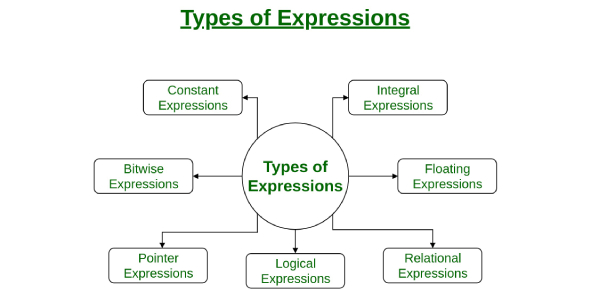# Expressions And Integers Test! Math Trivia Quiz

15 Questions | Total Attempts: 80SettingsHow well do you know expressions and integers? Would you be able to ACE this quiz? An integer is defined as a number that can be written out without a fractional component. An expression is a fixed combination of well-constructed symbols according to rules that depend on the context, and you can use symbols, figures, or equations. This quiz is all about expressions and integers. You will do great on this quiz.

• 1.
Sara and Kim together have  15 pencils.  Sara has 9  pencils. Mark and Kim together have 10 pencils.  How many pencils does Mark have?
• A.

5

• B.

34

• C.

8

• D.

4

• 2.
Steve has x dollars in his bank account.  He deposits \$10 each week for 3 weeks.  His bank balance now is \$70.  What is x? ( How much money did he have to start?)
• A.

\$60

• B.

\$20

• C.

\$40

• D.

\$50

• 3.
It is 19 degrees in Alaska at 9PM.  The temperature drops 23 degrees overnight but then rises 9 degrees by 9AM the following day.  What is the temperature at 9AM?
• A.

5 degrees

• B.

-5 degrees

• C.

13 degrees

• D.

-1 degree

• 4.
Lisa is in a submarine 87 feet below sea level.  Barb is hiking up a hill and is 125 feet above sea level.  Greg snorkeling 5 feet under water.  List their elevations in order least to greatest.
• A.

5 , 87 , 125

• B.

125 , 87 , 5

• C.

- 87 , -5 , 125

• D.

-5 , -87 , 125

• 5.
Find the value of  n in the equation below. 2n  +  10   =   20         n = _____
• A.

10

• B.

5

• C.

8

• D.

20

• 6.
Find the value of  n in the equation below. 15  +  n   +   20  =    45          n = _______
• A.

10

• B.

5

• C.

12

• D.

8

• 7.
Find the value of  n in the equation below. 12   x    23   x    9   x  n    x  56   =    0              n = _____
• A.

10

• B.

5

• C.

0

• D.

1

• 8.
Find the value of  n in each equation below. 3n   +    5  =    14            n=   ____________
• A.

1

• B.

3

• C.

4

• D.

2

• 9.
Find the next two  numbers in the pattern: -8   -6   -4   -2     ___      ___
• A.

-1 , 1

• B.

-1 , 0

• C.

0 , 2

• D.

0 , 1

• 10.
Find the next two numbers in this pattern: -15     -14      -12     -11      -9    ____     _____
• A.

-7 , -5

• B.

-8 , -6

• C.

-7 , -6

• D.

-8 , -7

• 11.
Find the next two numbers in this pattern: 25      20      21      16     17    ____     ____
• A.

12 , 13

• B.

13 , 14

• C.

18 , 19

• D.

21 , 20

• 12.
Find the next two numbers in this pattern: 100        85        70         55     _____     _____
• A.

45 , 30

• B.

50 , 40

• C.

40 , 30

• D.

40 , 25

• 13.
Find the next two numbers in this pattern:-20   -15    -10     -5     ___     ___
• A.

5 , 10

• B.

0 , 5

• C.

0 , 10

• D.

-10 , -5

• 14.
Brett got on an elevator and rode up 10 floors.  Next he rode down 7 floors.  After that he went up 4 floors.  Finally he went down 6 floors.  When he got off the elevator he was on the 11th floor.  What floor was he on when he started his ride?
• A.

8th floor

• B.

9th floor

• C.

10th floor

• D.

12th floor

• 15.
A good way to check and see if you have correctly found the value of an unknown number in an expression is to plug your answer into the expression and see if it is true.
• A.

True

• B.

False

Related TopicsBack to top